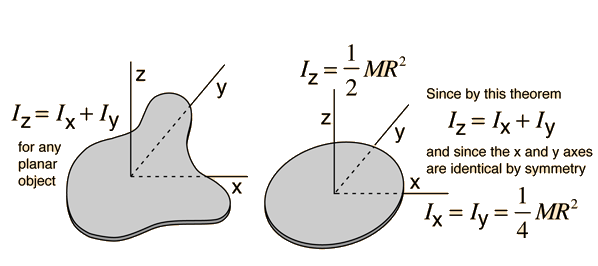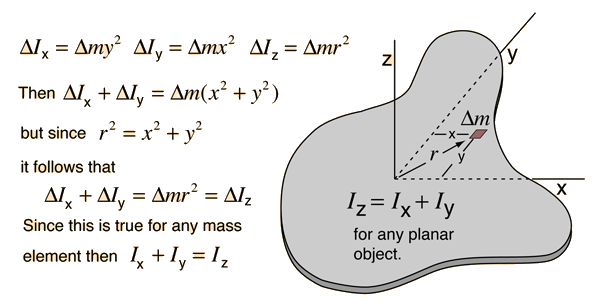Perpendicular Axis Theorem

For a planar object, the moment of inertia about an axis perpendicular to the plane is the sum of the moments of inertia of two perpendicular axes through the same point in the plane of the object. The utility of this theorem goes beyond that of calculating moments of strictly planar objects. It is a valuable tool in the building up of the moments of inertia of three dimensional objects such as cylinders by breaking them up into planar disks and summing the moments of inertia of the composite disks.Show the development of the relationship.

Index

Moment of inertia concepts

 HyperPhysics***** Mechanics R Nave
Go Back

Perpendicular Axis Theorem

The perpendicular axis theorem for planar objects can be demonstrated by looking at the contribution to the three axis moments of inertia from an arbitrary mass element. From the point mass moment, the contributions to each of the axis moments of inertia areIndex

Moment of inertia concepts

 HyperPhysics***** Mechanics R Nave
Go Back